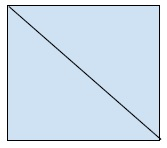# Area of a square from diagonal length in C++

The area of a figure is the extent of the figure in two-dimensional plane.

Square is a quadrilateral with all its sides equal and all internal angles are right angles.

Diagonal of a polygon is the line joining two sides that are not adjacent to each other.ac and bd are the diagonal of the square abcd.

In this problem, we are given the length of diagonals of a square and we have to find are of the square.

Now in triangle abc,

ac2 = bc2 + ab2
d2 = a2 + a2
d = sqrt(2*a2)
d2 /2 = a2

And we know are of square = a * a.

Therefore,

area = d2 /2

Using this formula we can find the area of a square when the length of diagonal is given,

## Example

Live Demo

#include<iostream>
#include<math.h>
using namespace std;
int main(){
double d = 10;
double area = (d * d)/2.0;
cout<<"Area of square of diagonal "<<d<<" is "<<area;
return 0;
}

## Output

area of square of diagonal 10 is 50